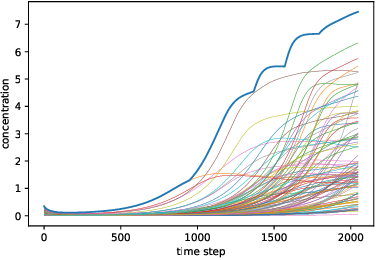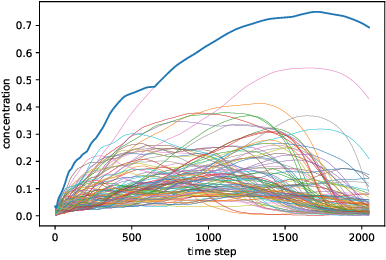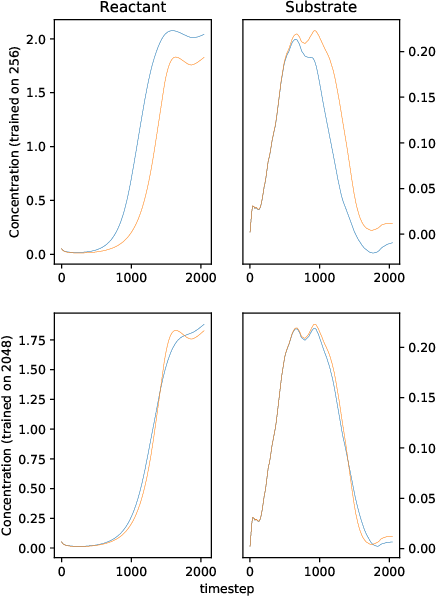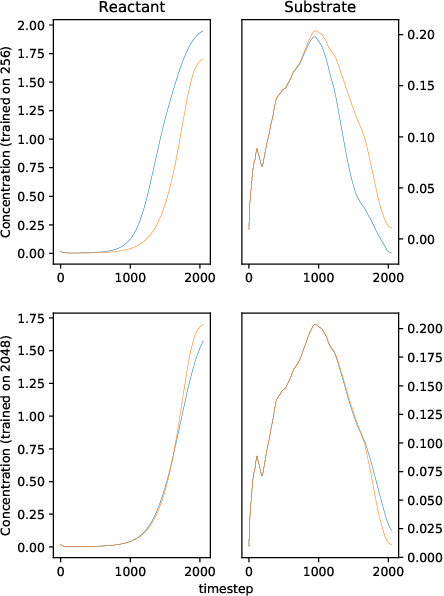We solve a system of ordinary differential equations with an unknown functional form of a sink (reaction rate) term. We assume that the measurements (time series) of state variables are partially available, and we use recurrent neural network to "learn" the reaction rate from this data. This is achieved by including a discretized ordinary differential equations as part of a recurrent neural network training problem. We extend TensorFlow's recurrent neural network architecture to create a simple but scalable and effective solver for the unknown functions, and apply it to a fedbatch bioreactor simulation problem. Use of techniques from recent deep learning literature enables training of functions with behavior manifesting over thousands of time steps. Our networks are structurally similar to recurrent neural networks, but differences in design and function require modifications to the conventional wisdom about training such networks.

* 19 pages, 8 figuresClick To Get Code For Paper